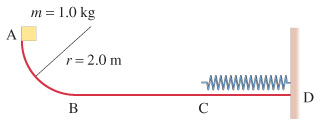# Problem: Consider the track shown in the figure. The section AB is one quadrant of a circle of radius 2.0 m and is frictionless. B to C is a horizontal span 3.1 m long with a coefficient of kinetic friction exttip{mu_{ m k}}{mu} = 0.24. The section CD under the spring is frictionless. A block of mass 1.0 kg is released from rest at A. After sliding on the track, it compresses the spring by 0.30 m . Determine the velocity of the block at point B.Determine the thermal energy produced as the block slides from B to C.Determine the velocity of the block at point C.Determine the stiffness constant k for the spring.

###### FREE Expert Solution
99% (451 ratings)
###### Problem Details

Consider the track shown in the figure. The section AB is one quadrant of a circle of radius 2.0 m and is frictionless. B to C is a horizontal span 3.1 m long with a coefficient of kinetic friction = 0.24. The section CD under the spring is frictionless. A block of mass 1.0 kg is released from rest at A. After sliding on the track, it compresses the spring by 0.30 m .Determine the velocity of the block at point B.

Determine the thermal energy produced as the block slides from B to C.

Determine the velocity of the block at point C.

Determine the stiffness constant k for the spring.

Frequently Asked Questions

What scientific concept do you need to know in order to solve this problem?

Our tutors have indicated that to solve this problem you will need to apply the Springs & Elastic Potential Energy concept. You can view video lessons to learn Springs & Elastic Potential Energy. Or if you need more Springs & Elastic Potential Energy practice, you can also practice Springs & Elastic Potential Energy practice problems.